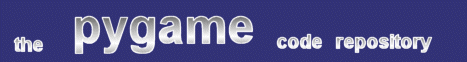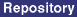### Title: 2d Vector Class

Author: Chris Wood (http://grace.2ya.com)
Submission date: September 20, 2002

Description: A simple 2d vector class

pygame version required: None
SDL version required: None
Python version required: 2.0

Comments: Here's a small class that provides the basics of 2d vector math. Adding other functions like vector multiplication and dot product calculation should be simple if you follow the outline of this class.

```
"""
vector.py
A simple physics 2D vector class
Licence: Public Domain
Author: Chris Wood (http://grace.2ya.com)
20 September 2002

Any game involving 2D physics could do with a class similar to this one. This
class is as fast as possible while still providing all the functionality you
would commonly need.

Note that storing the vector as cartesian coordinates (rather than polar or
both) makes for faster for vector addition. It is assumed that you won't often
need the polar coordinates.
"""

from math import sin, cos, atan, hypot

# The quick way to know how big the pie is
PI = 3.141592653589793238462643383
TwoPI = PI * 2.0
HalfPI = PI * 0.5
OneAndHalfPI = PI * 1.5

# new math function
def direction(x, y):
"""Return the direction component of a vector (in radians), given
cartesian coordinates.
"""
if x > 0:
if y >= 0:
return atan(y / x)
else:
return atan(y / x) + TwoPI
elif x == 0:
if y > 0:
return HalfPI
elif y == 0:
return 0
else:
return OneAndHalfPI
else:
return atan(y / x) + PI

class Vector:
"""Store a vector in cartesian coordinates."""

def __init__(self, f = 0.0, mag = 0.0):
"""Create a Vector, given polar coordinates."""
# Calculate cartesian coordinates
self.x = mag * cos(f)
self.y = mag * sin(f)

"""Add b to self, where b is another Vector."""
self.x += b.x
self.y += b.y

"""Return the direction of the Vector in radians."""
return direction(self.x, self.y)

def mag(self):
"""Return the magnitude of the Vector."""
return hypot(self.x, self.y)

```
 From: Dave Beach Date: January 31, 2004 17:17 GMT This class could be made faster for Python 2.3 with the following small changes: class Vector(object): """Store a vector in cartesian coordinates.""" __slots__ = ('x', 'y')

 From: Anonymous Date: February 26, 2004 12:11 GMT ( from quick-reference site ) Note: According to recent discussions, the real purpose of slots seems still unclear (optimization?), and their use should probably be discouraged.

 Feedback